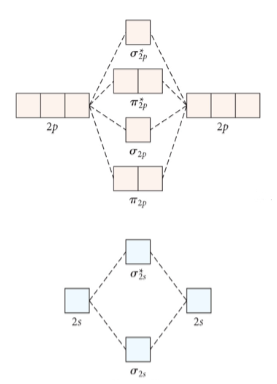# Problem: Given the molecular orbital diagram below, determine the bond order for nitrogen gas, N2 A. 0               B. 1                    C. 2                  D. 3                E. 4

🤓 Based on our data, we think this question is relevant for Professor DeSimone's class at UA.

###### Problem Details

Given the molecular orbital diagram below, determine the bond order for nitrogen gas, N2

A. 0

B. 1

C. 2

D. 3

E. 4What scientific concept do you need to know in order to solve this problem?

Our tutors have indicated that to solve this problem you will need to apply the Bond Order concept. If you need more Bond Order practice, you can also practice Bond Order practice problems.

What is the difficulty of this problem?

Our tutors rated the difficulty ofGiven the molecular orbital diagram below, determine the bon...as high difficulty.

How long does this problem take to solve?

Our expert Chemistry tutor, Dasha took 3 minutes and 2 seconds to solve this problem. You can follow their steps in the video explanation above.

What professor is this problem relevant for?

Based on our data, we think this problem is relevant for Professor DeSimone's class at UA.